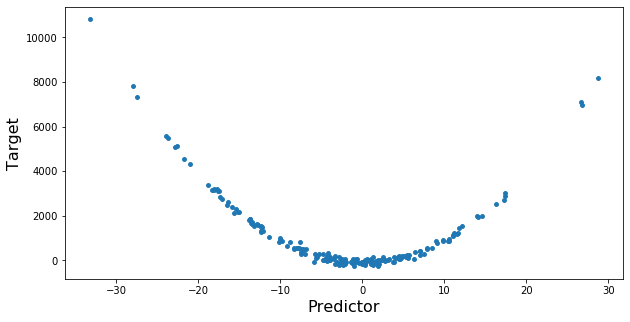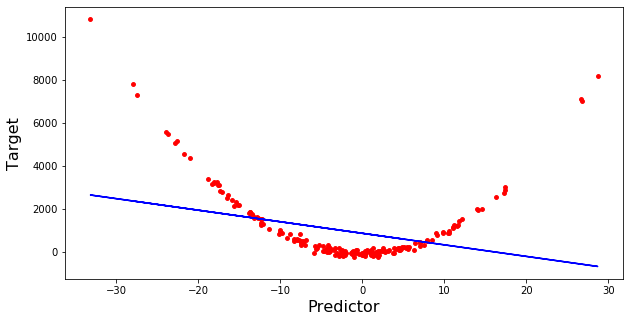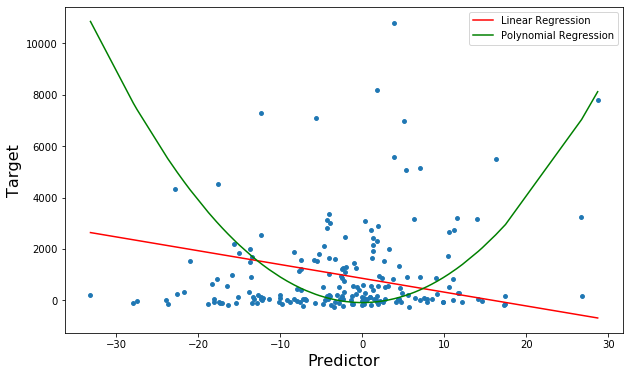0%
Polynomial Regression Full Implementation.
You will use use the most basic and the Polynomial model to predict results.

# Polynomial Regression

In this notebook, we learn how to use scikit-learn for Polynomial regression. We download a dataset that is related to fuel consumption and Carbon dioxide emission of cars. Then, we split our data into training and test sets, create a model using training set, evaluate our model using test set, and finally use model to predict unknown value.

### Importing Needed packages¶

In :
import matplotlib.pyplot as plt
import pandas as pd
import pylab as pl
import numpy as np
%matplotlib inline


## Creating the data¶

Sometimes, the trend of data is not really linear, and looks curvy. In this case we can use Polynomial regression methods. In fact, many different regressions exist that can be used to fit whatever the dataset looks like, such as quadratic, cubic, and so on, and it can go on and on to infinite degrees.

In essence, we can call all of these, polynomial regression, where the relationship between the independent variable x and the dependent variable y is modeled as an nth degree polynomial in x. Lets say you want to have a polynomial regression (let's make 2 degree polynomial):

$y = b + \theta_1 x + \theta_2 x^2$

Now, the question is: how we can fit our data on this equation while we have only x values, such as Engine Size? Well, we can create a few additional features: 1, $x$, and $x^2$.

PloynomialFeatures() function in Scikit-learn library, drives a new feature sets from the original feature set. That is, a matrix will be generated consisting of all polynomial combinations of the features with degree less than or equal to the specified degree. For example, lets say the original feature set has only one feature, ENGINESIZE. Now, if we select the degree of the polynomial to be 2, then it generates 3 features, degree=0, degree=1 and degree=2:

In :
x = 10*np.random.normal(0,1,200)
y = 10*(x**2)+np.random.normal(-100, 100,200)
x = x.reshape(-1,1) #-1 in the x value will retain the x shape and will change the y shape only
y = y.reshape(-1,1)
print(x.shape,'\n', y.shape)

# plotting dataset
plt.figure(figsize=(10,5))
plt.scatter(x,y,s=15)
plt.xlabel('Predictor',fontsize=16)
plt.ylabel('Target',fontsize=16)
plt.show()

(200, 1)
(200, 1)In :
from sklearn.linear_model import LinearRegression
linreg = LinearRegression()
linreg.fit(x,y)

#plot the results

plt.figure(figsize=(10,5))
plt.scatter(x,y,s=15, color = 'red')
plt.plot(x, linreg.predict(x), color = 'blue')
plt.xlabel('Predictor',fontsize=16)
plt.ylabel('Target',fontsize=16)
plt.show()In :
from sklearn.metrics import mean_squared_error
print('RMSE for Linear Regression=>',np.sqrt(mean_squared_error(y,linreg.predict(x))))

RMSE for Linear Regression=> 1635.1889668724025


## Now, let’s try polynomial regression.¶

In :
# importing libraries for polynomial transform
#from sklearn.preprocessing import PolynomialFeatures

#polynomial_features= PolynomialFeatures(degree=2)
#x_poly = polynomial_features.fit_transform(x)

#model = LinearRegression()
#model.fit(x_poly, y)
#y_poly_pred = model.predict(x_poly)

# importing libraries for polynomial transform
from sklearn.preprocessing import PolynomialFeatures
# for creating pipeline
from sklearn.pipeline import Pipeline
# creating pipeline and fitting it on data
Input=[('polynomial',PolynomialFeatures(degree=2)),('modal',LinearRegression())]
pipe=Pipeline(Input)
pipe.fit(x,y)

Out:
Pipeline(memory=None,
steps=[('polynomial', PolynomialFeatures(degree=2, include_bias=True, interaction_only=False)), ('modal', LinearRegression(copy_X=True, fit_intercept=True, n_jobs=None,
normalize=False))])

## Fitting Our model¶

In :
#plotting predictions
import operator

plt.figure(figsize=(10,6))
plt.scatter(x, y, s=15)

# sort the values of x before line plot
sort_axis = operator.itemgetter(0)
sorted_zip = sorted(zip(x,y_poly_pred), key=sort_axis)
x, y_poly_pred = zip(*sorted_zip)

plt.plot(x,linreg.predict(x),color='r',label='Linear Regression')
plt.plot(x, y_poly_pred, color='g',label='Polynomial Regression')
plt.xlabel('Predictor',fontsize=16)
plt.ylabel('Target',fontsize=16)
plt.legend()
plt.show()In :
print('RMSE for Polynomial Regression=>',np.sqrt(mean_squared_error(y,poly_pred)))

RMSE for Polynomial Regression=> 1690.2443340832644


## But what if we have more than one predictor?

For 2 predictors, the equation of the polynomial regression becomes:

two degree polynomial regression

where,

      Y is the target,

x1, x2 are the predictors,

𝜃0 is the bias,

and, 𝜃1, 𝜃2, 𝜃3, 𝜃4, and 𝜃5 are the weights in the regression equation



For n predictors, the equation includes all the possible combinations of different order polynomials. This is known as Multi-dimensional Polynomial Regression.

But, there is a major issue with multi-dimensional Polynomial Regression – multicollinearity. Multicollinearity is the interdependence between the predictors in a multiple dimensional regression problem. This restricts the model from fitting properly on the dataset.

## Advantages of using Polynomial Regression:¶

• Broad range of function can be fit under it.
• Polynomial basically fits wide range of curvature.
• Polynomial provides the best approximation of the relationship between dependent and independent variable.

## Disadvantages of using Polynomial Regression¶

• These are too sensitive to the outliers.
• The presence of one or two outliers in the data can seriously affect the results of a nonlinear analysis.
• In addition there are unfortunately fewer model validation tools for the detection of outliers in nonlinear regression than there are for linear regression.

## Practice

Try to use a polynomial regression with the dataset but this time with degree three (cubic). Does it result in better accuracy?

In [ ]:
# write your code here